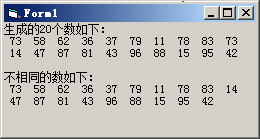﻿ 在一个数列中，删除其中的重复数，使得数列只保留不同的数。---- 第六章 数组 新编Visual Basic程序设计教程 陈斌 著 - 何老师的济亨网

# 在一个数列中，删除其中的重复数，使得数列只保留不同的数。---- 第六章 数组 新编Visual Basic程序设计教程 陈斌 著

###### 2017-06-01 09:14:57  分类: VB程序题  参与: AddViewCount(162)人

4. 在一个数列中，删除其中的重复数，使得数列只保留不同的数。

Option Base 1
Private Sub Form_Click()
Dim a() As Integer, n As Integer
Dim i As Integer, j As Integer, k As Integer
n = InputBox("请输入数据个数：")
ReDim a(n)
Cls
Print "生成的" & n & "个数如下："
For i = 1 To n
a(i) = Int(Rnd * 90) + 10
Print a(i);
If i Mod 10 = 0 Then Print '每输出10个数据就换行
Next i

i = 1
Do While i <= n - 1
j = i + 1
Do While j <= n
If a(i) = a(j) Then '发现相同的数
Text1.Text = Text1.Text & " " + Str(a(i))
For k = j To n - 1
a(k) = a(k + 1) '发现有相同的数，就把数组元素后面的数组元素依次前移
Next k
n = n - 1
ReDim Preserve a(n) '动态数组定义
Else
j = j + 1
End If
Loop
i = i + 1
Loop

Print
Print "不相同的数如下："
For i = 1 To n
Print a(i);
If i Mod 10 = 0 Then Print
Next i
Print
End Sub---- 新编Visual Basic程序设计教程  答案 陈斌  著

<< 上一篇下一篇 >>